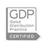# International Shipping Rates

## Use our tables to calculate the cost of your shipments by air, truck and sea

##### REQUESTS FOR FURTHER INFORMATION

To get in touch with us please fill-in the following form.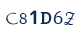We collect your personal data for marketing purpose.

We collect your data for users profiling.

 Conversion factors: pounds to kilograms: multiply pounds by 0.45359 inches to centimeters: multiply inches by 2.54 feet to meters: multiply feet by 0.3048 yards to meters: multiply yards by 0.9144 kilograms to pounds: multiply kilograms by 2.20462 centimeters to inches: multiply centimeters by 0.3937 meters to feet: multiply meters by 3.2808 meters to yards: multiply meters by 1.0936
 Volume weight The weight you will be charged for a shipment will be either the actual weight or the volume weight (whichever is higher). To calculate the volume weight see the following table:
Mode Factor Conversion How to calculate
Air freight "1/6" 1 cubic meter = 166.6 kg length x width x height / 6000
Truck "1/3" 1 cubic meter = 330 kg length x width x height / 3000
Ocean "1/1" 1 cubic meter = 1000 kg no data
 Example for an air freight shipment: 3 boxes Actual weight = 100 kg Dimensions: 3 cartons each - 100 cm (length) x 72 cm (width) x 120 cm (height) Volume weight calculation: 100 x 72 x 120 = 864,000 864,000 x 3 (number of boxes) = 2,592,000 2,592,000 divided by 6000 = 432 kg Volume weight = chargeable weight = 432 kg
 The charges for this shipment would be based on 432 kg. (and not 100 kg.)
##### Certifications
•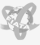•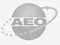•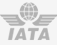•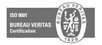•